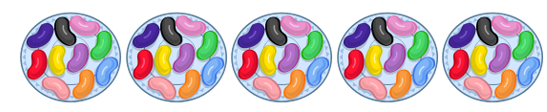Mathematics
Easy

Question

# Count the jelly beans by 10s.## 50603080Hint:

## The correct answer is: 50

### Here, we have to count the jelly beans by 10s.Total number of groups = 5 .Start by 10s = 10 , 20 , 30 , 40 , 50 .Therefore, 5 tens = 50 .Hence, the correct option is (c).

Counting numbers are the set of numbers that we used to count anything.

### Related Questions to study#### With Turito Foundation.#### Get an Expert Advice From Turito.